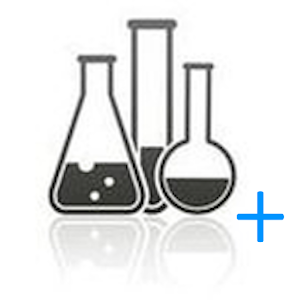Nederlands

We bieden dagelijks GRATIS gelicenseerde Android apps en games aan, die je normaal zou moeten kopen.\$1.49
VERSTREKEN

# Android Giveaway of the Day - AlcoholCalculator+

Calculator for alcohol dilution.
\$1.49 VERSTREKEN
Waarderingscijfer: 1 (100%) 0 (0%)

Deze giveaway is verlopen. AlcoholCalculator+ is nu beschikbaar op de normale manier.

Calculator for alcohol dilution (calculation of the required amount of alcohol and water to obtain an alcohol mixture of the desired strength).
The calculation is made taking into account the contraction (change in the total volume when mixing two liquids).
+ calculation of mixing of two/three solutions of varying degree of alcohol content.
+ calculation of dilution by weight.
+ calculation of the alcohol content of the solution in the cube and the steam temperature in the cube.
+ calculation of density change from temperature.
+ calculation of alcohol content from the boiling point, taking into account ATM.pressures.
+ calculation of mixing cold and hot water to obtain the desired temperature and volume.
+ timer calculation selection for a given time (for example, the selection of goals in ml / hour)
+ tab for calculating the dissolution of sugar in water.
+Added the heating POWER CALCULATION tab

### Ontwikkelaar:

Корабельников Алексей

### Categorie:

Books & Reference

2.0.14

1.5M

Everyone

4.0 and up

## Reactie op AlcoholCalculator+

Thank you for voting!